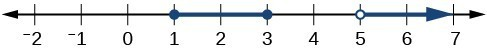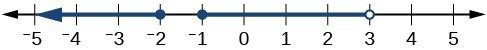## 1.1 – Interval and Set-Builder Notation

### Learning Objectives

• (1.1.1) – Represent inequalities on a number line
• (1.1.2) – Graphing an Inequality
• (1.1.3) – Represent inequalities using interval notation
• (1.1.4) – Set-builder notation

Sometimes there is a range of possible values to describe a situation. When you see a sign that says “Speed Limit 25,” you know that it doesn’t mean that you have to drive exactly at a speed of 25 miles per hour (mph). This sign means that you are not supposed to go faster than 25 mph, but there are many legal speeds you could drive, such as 22 mph, 24.5 mph or 19 mph. In a situation like this, which has more than one acceptable value, inequalities are used to represent the situation rather than equations.

Solving multi-step inequalities is very similar to solving equations—what you do to one side you need to do to the other side in order to maintain the “balance” of the inequality. The Properties of Inequality can help you understand how to add, subtract, multiply, or divide within an inequality.

# (1.1.1) – Represent inequalities on a number line

First, let’s define some important terminology. An inequality is a mathematical statement that compares two expressions using the ideas of greater than or less than. Special symbols are used in these statements. When you read an inequality, read it from left to right—just like reading text on a page. In algebra, inequalities are used to describe large sets of solutions. Sometimes there are an infinite amount of numbers that will satisfy an inequality, so rather than try to list off an infinite amount of numbers, we have developed some ways to describe very large lists in succinct ways.

The first way you are probably familiar with—the basic inequality. For example:

• ${x}\lt{9}$ indicates the list of numbers that are less than 9. Would you rather write ${x}\lt{9}$ or try to list all the possible numbers that are less than 9? (hopefully, your answer is no)
• $-5\le{t}$ indicates all the numbers that are greater than or equal to $-5$.

Note how placing the variable on the left or right of the inequality sign can change whether you are looking for greater than or less than.

For example:

• $x\lt5$ means all the real numbers that are less than 5, whereas;
• $5\lt{x}$ means that 5 is less than x, or we could rewrite this with the x on the left: $x\gt{5}$ note how the inequality is still pointing the same direction relative to x. This statement represents all the real numbers that are greater than 5, which is easier to interpret than 5 is less than x.

The second way is with a graph using the number line:And the third way is with an interval.

We will explore the second and third ways in depth in this section. Again, those three ways to write solutions to inequalities are:

• an inequality
• an interval
• a graph

### Inequality Signs

The box below shows the symbol, meaning, and an example for each inequality sign. Sometimes it’s easy to get tangled up in inequalities, just remember to read them from left to right.

Symbol Words Example
$\neq$ not equal to ${2}\neq{8}$, 2 is not equal to 8.
$\gt$ greater than ${5}\gt{1}$, 5 is greater than 1
$\lt$ less than ${2}\lt{11}$, 2 is less than 11
$\geq$ greater than or equal to ${4}\geq{ 4}$, 4 is greater than or equal to 4
$\leq$ less than or equal to ${7}\leq{9}$, 7 is less than or equal to 9

The inequality $x>y$ can also be written as ${y}<{x}$. The sides of any inequality can be switched as long as the inequality symbol between them is also reversed.

# (1.1.2) – Graphing an Inequality

Inequalities can also be graphed on a number line. Below are three examples of inequalities and their graphs.  Graphs are a very helpful way to visualize information – especially when that information represents an infinite list of numbers!

$x\leq -4$. This translates to all the real numbers on a number line that are less than or equal to 4.${x}\geq{-3}$. This translates to all the real numbers on the number line that are greater than or equal to -3.Each of these graphs begins with a circle—either an open or closed (shaded) circle. This point is often called the end point of the solution. A closed, or shaded, circle is used to represent the inequalities greater than or equal to $\displaystyle \left(\geq\right)$ or less than or equal to $\displaystyle \left(\leq\right)$. The point is part of the solution. An open circle is used for greater than (>) or less than (<). The point is not part of the solution.

The graph then extends endlessly in one direction. This is shown by a line with an arrow at the end. For example, notice that for the graph of $\displaystyle x\geq -3$ shown above, the end point is $−3$, represented with a closed circle since the inequality is greater than or equal to $−3$. The blue line is drawn to the right on the number line because the values in this area are greater than $−3$. The arrow at the end indicates that the solutions continue infinitely.

### Example

Graph the inequality $x\ge 4$

This video shows an example of how to draw the graph of an inequality.

### Example

Write and inequality describing all the real numbers on the number line that are less than 2, then draw the corresponding graph.

# (1.1.3) – Represent inequalities using interval notation

Another commonly used, and arguably the most concise, method for describing inequalities and solutions to inequalities is called interval notation. With this convention, sets are built with parentheses or brackets, each having a distinct meaning. The solutions to $x\geq 4$ are represented as $\left[4,\infty \right)$. This method is widely used and will be present in other math courses you may take.

The main concept to remember is that parentheses represent solutions greater or less than the number, and brackets represent solutions that are greater than or equal to or less than or equal to the number. Use parentheses to represent infinity or negative infinity, since positive and negative infinity are not numbers in the usual sense of the word and, therefore, cannot be “equaled.” A few examples of an interval, or a set of numbers in which a solution falls, are $\left[-2,6\right)$, or all numbers between $-2$ and $6$, including $-2$, but not including $6$; $\left(-1,0\right)$, all real numbers between, but not including $-1$ and $0$; and $\left(-\infty,1\right]$, all real numbers less than and including $1$. The table below outlines the possibilities. Remember to read inequalities from left to right, just like text.

The table below describes all the possible inequalities that can occur and how to write them using interval notation, where a and b are real numbers.

Inequality Words Interval Notation
${a}\lt{x}\lt{ b}$ all real numbers between a and b, not including a and b $\left(a,b\right)$
${x}\gt{a}$ All real numbers greater than a, but not including a $\left(a,\infty \right)$
${x}\lt{b}$ All real numbers less than b, but not including b $\left(-\infty ,b\right)$
${x}\ge{a}$ All real numbers greater than a, including a $\left[a,\infty \right)$
${x}\le{b}$ All real numbers less than b, including b $\left(-\infty ,b\right]$
${a}\le{x}\lt{ b}$ All real numbers between a and b, including a $\left[a,b\right)$
${a}\lt{x}\le{ b}$ All real numbers between a and b, including b $\left(a,b\right]$
${a}\le{x}\le{ b}$ All real numbers between a and b, including a and b $\left[a,b\right]$
${x}\lt{a}\text{ or }{x}\gt{ b}$ All real numbers less than a or greater than b $\left(-\infty ,a\right)\cup \left(b,\infty \right)$
All real numbers All real numbers $\left(-\infty ,\infty \right)$

### Example

Describe the inequality $x\ge 4$ using interval notation

### Example

Use interval notation to indicate all real numbers greater than or equal to $-2$.

### Example

Use interval notation to indicate all real numbers between $-3$ and $5$, including $5$.

In the previous examples you were given an inequality or a description of one with words and asked to draw the corresponding graph and write the interval. In this example you are given an interval and asked to write the inequality and draw the graph.

Given $\left(-\infty,10\right)$, write the associated inequality and draw the graph.

In the box below, write down whether you think it will be easier to draw the graph first or write the inequality first.

In the following video, you will see examples of how to write inequalities in the three ways presented here: as an inequality, in interval notation, and with a graph.

# (1.1.4) – Set-builder notation

Another useful tool in specifying subsets of the real line is set-builder notation.

### A General Note: Set-Builder Notation and Interval Notation

Set-builder notation is a method of specifying a set of elements that satisfy a certain condition. It takes the form $\left\{x|\text{statement about }x\right\}$ which is read as, “the set of all $x$ such that the statement about $x$ is true.” For example,

$\left\{x|4<x\le 12\right\}$

Interval notation is a way of describing sets that include all real numbers between a lower limit that may or may not be included and an upper limit that may or may not be included. The endpoint values are listed between brackets or parentheses. A square bracket indicates inclusion in the set, and a parenthesis indicates exclusion from the set. For example,

$\left(4,12\right]$

### Example: Describing Sets on the Real-Number Line

Describe the intervals of values shown below using inequality notation, set-builder notation, and interval notation.### Try It

Given the graph below, specify the graphed set in

1. words
2. set-builder notation
3. interval notationThis video describes how to use interval notation to describe a set.

This video describes how to use Set-Builder notation to describe a set.